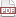### Interrater agreement via entropy

26 September 13. [link]PDF version

Last time, I wrote about interrater agreement, in which we seek a measure of the agreement rate between two people categorizing the same set of observations. We want it to be complexity-adjusted, because two raters putting data into two categories can achieve 50% agreement by flipping coins, while 50% agreement on a task with a hundred fine-distinction categories may be an impressively high agreement rate.

The literature I described did this via model-based means: set up a scenario where categories are assigned at random, and compare the observed agreement rate with that at-random rate. The problem is that this opens the door to a dozen different means of defining the at-random scenario. I gave the distinction between Scott's Pi and Cohen's Kappa as an initial example, but the literature goes on: what if the task is putting a continuous observation into discrete bins, so if rater one picked 50, rater two is very likely to pick 49, 50, or 51? What if one rater abuses the other category, but the raters are otherwise always in agreement? Would it be a reasonable model to presume that raters are accurate $k$% of the time, but flub and draw a random category $1-k$% of the time? Andrés and Marzo (2004) propose this model.

When I first encountered this literature, it brought to mind entropy, which is often informally billed as a measure of the complexity of the system. It's also billed as a measure of the information in a system: where entropy is large (e.g., a hundred equiprobable bins), then each new draw provides new information; where entropy is near zero (e.g., only one category, or 90% of type A and 10% of type B), we know what the next draw will probably be before seeing it.

The entropy of a probability mass function with $i$ categories is defined as as $H =\sum_i -p_i \log_2(p_i)$. If a bin has $p_i=0$, we define that bin to have zero contribution to the entropy (which is the correct limit of $p_i \log_2(p_i)$ as $p_i\to 0$). Notice that the log of a number between zero and one is negative (or zero if $p_i=1$), so the negation is positive (or zero).

Entropists think of information as a real quantity, which can be measured like anything else. Its unit is the bit, which is short for binary digit. Notably, if there are two streams, there is a total entropy, and some amount of mutual information between them. Let $I(X, Y)$ be the mutual information; If I know the streams are in sync, then seeing one observation tells me exactly what the other stream is saying, so we should have that $H(X) = H(Y) = I(X, Y)$. If the streams are independent (knowing $X$ gives no information about $Y$), then we should have $I(X, Y)=0$.

The formula for mutual information given a set of categories is

$$I(X, Y) = \sum_j \sum_i p_{ij} \log_2\left(\frac{p_{ij}}{p_{\cdot{}j}p_{i\cdot}}\right),$$

where $p_{\cdot{}j}$ is the odds of category $j$ regardless of $i$, and similarly for $p_{i\cdot}$. We are looking for information in agreement, so define $IA(X, Y)$ to be this sum only over indices where $i=j$, throwing out those elements of the sum where $i\neq j$.

The raw percent agreement is $P_o\equiv$ (count of observations in agreement)/(total count of observations). By analogy, define

$$P_I(X, Y)\equiv \frac{IA(X, Y)}{(H(X)+H(Y))/2},$$

that is, the (information in agreement)/(mean total individual information).

This is a sensible measure of interrater agreement:

• It is one when it should be (full agreement).

• It is zero when it should be (agreement equal to the random rate, or no agreement at all). In the no-agreement case, Cohen's Kappa is negative. Exercise: prove that the range of Cohen's Kappa is from $-1/(B-1)$ to $1$, where $B$ is the number of bins.

• It is complexity-adjusting in the expected ways.

• If you are comfortable with the concept of measuring information as bits of entropy, then it is a simple and natural fraction of agreement-to-total, akin to $P_o$.

• It is model-independent: we used information accounting identities which work for any pair of distributions, so we entirely sidestep the debate about how to define agreement at random.

I wrote up $P_I$ and its properties in detail in this Journal of Official Statistics paper. I hate to be immodest, but I really do like $P_I$ better than the alternatives. As I discuss in the paper, there are natural extensions to ordered categories (by redefining $IA(X, Y)$ to accommodate near-misses) and to multiple raters (by extending the numerator to include information in agreement across all pairs and the denominator to mean total entropy for all pairs). I think that having a system that sidesteps the problem of developing a baseline is a real win. The payoff comes when writing the paper using the measure, because using a statistic designed around a baseline at-random model requires a paragraph or two explaining why the situation at hand is compatible with the assumptions underlying that model, and explaining why those assumptions are more appropriate than those for the models underlying other measures referees might be used to. Meanwhile, it is theoretically valid to measure the mutual information for any two streams of data.

Last time, I presented a code example that gave some code to calculate $P_I$ from the confusion matrix, and now would be a good time to remind you that all the code is available on GitHub. If you're not a C user, you should have no problem translating the C code into your favorite language. You are welcome to try it out and see if it does what an intercoder agreement index should.